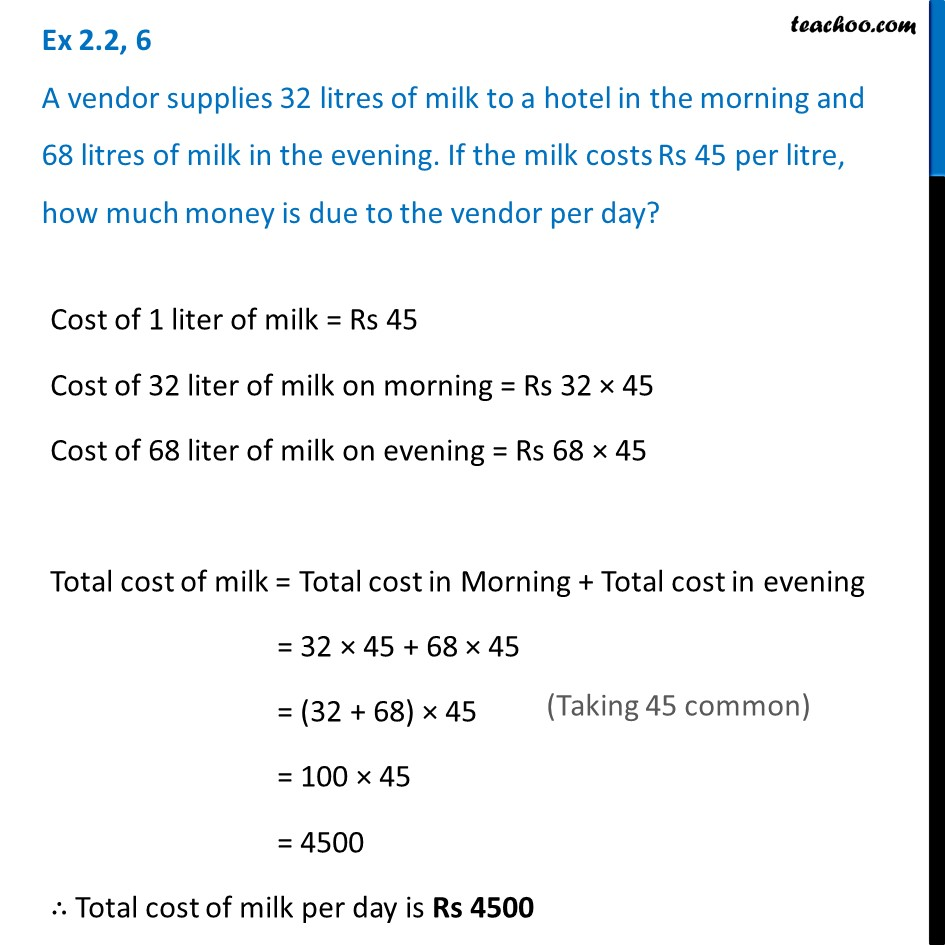Sum, Multiplication, Division of Whole Numbers

Chapter 2 Class 6 Whole Numbers
Serial order wiseLearn in your speed, with individual attention - Teachoo Maths 1-on-1 Class

### Transcript

Question 6 A vendor supplies 32 litres of milk to a hotel in the morning and 68 litres of milk in the evening. If the milk costs Rs 45 per litre, how much money is due to the vendor per day? Cost of 1 liter of milk = Rs 45 Cost of 32 liter of milk on morning = Rs 32 × 45 Cost of 68 liter of milk on evening = Rs 68 × 45 Total cost of milk = Total cost in Morning + Total cost in evening = 32 × 45 + 68 × 45 = (32 + 68) × 45 (Taking 45 common) = 100 × 45 = 4500 ∴ Total cost of milk per day is Rs 4500